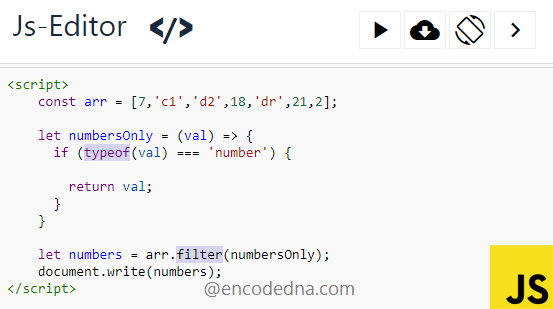﻿ How to filter out only Numbers in an Array using JavaScript

# How to filter out only Numbers in an Array using JavaScript

An array in JavaScript can hold different types of value in a single variable. The value can be a string or a number. Sometimes you might have to separate the numbers and strings from the array. I’ll show how easily you can filter out numbers in an array using JavaScript.Here’s an array, which has number and string values. I only want to filter out the numbers from the array.

let arr = [7,'c1','d2',18,'dr',21,2];

In JavaScript, you can use the typeof() method to determine whether a value is number or a string.

The typeof() method takes a parameter in the form of value. For example,

typeof(val)

Now, to check whether the given value is number, you can do something like this.

```if (typeof(val) === 'number') {
// … do something
}```

However, if you are extracting values from an array to get only the numbers, you need an iterator method like the .filter() or .map() methods. I am using the .filter() method here.

```<script>
var arr = [7, 'c1', 'd2', 18, 'dr', 21, 2];
var numbers = arr.filter(numbersOnly);

document.write(numbers);

function numbersOnly(value) {
if (typeof (value) === 'number') {
return value;
}
}
</script>```

I have explained about the .filter() method here.

The .filter() calls a functions (numberOnly), where I am checking the value type and return the value if it is a number. There’s no need to run an extra loop to get the values in the array. The .filter() method will take care of it and that’s why it is called the iterator method.

People who read this also read this: How to get only texts from array values in JavaScript

## Using ES6 features

Use this example if you are using ES6 features in your application.

```<script>
const arr = [7,'c1','d2',18,'dr',21,2];

let numbersOnly = (val) => {
if (typeof(val) === 'number') {      // replace number with string to get only string values in an array.
return val;
}
}

let numbers = arr.filter(numbersOnly);
document.write(numbers);
</script>```

Simple isn’t it. Now, if you want you can filter out only string values from an array. The method is same, except use string instead of number. Like this,

```if (typeof(val) === 'string') {
return val;
}```

Well, that’s it. Thanks for reading.